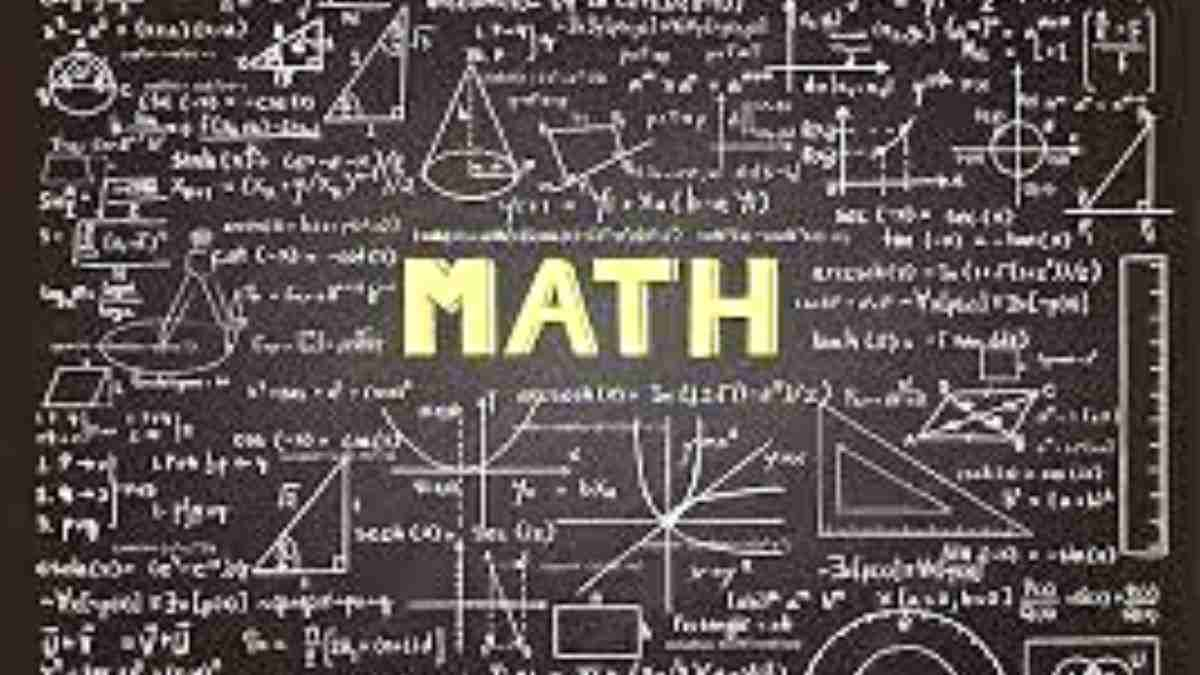# Who said math is tough? Try these fun math riddles!

Math is an interesting subject. Don’t believe us? Try these math riddles.What makes math the most hated subject? Well, the fact that it is super tough is what makes the subject undesirable by many students and adults alike.

However, today we can change your perception of the subject. How? With these math riddles.

### MATH RIDDLE 1:

If you multiply me by any other number, the answer will always remain the same. What number am I?

### MATH RIDDLE 2:

Which is correct: 18 plus 19 is 36 or, 18 plus 19 are 36?

### MATH RIDDLE 3:

What are the next three numbers in this pattern? 18, 21, 24, 27, 30, 33, 36, ______.

### MATH RIDDLE 4:

If Albert is 90 centimeters plus half his height, how tall is he?

### MATH RIDDLE 5:

If it took 7 people 10 hours to build the doghouse, how long would it take 15 people to build the same doghouse?

Finding the hidden tiger is as hard as escaping it! Will you dare to find it?

MATH RIDDLE 1:

If you multiply me by any other number, the answer will always remain the same. What number am I?

MATH RIDDLE 2:

Which is correct: 18 plus 19 is 36 or, 18 plus 19 are 36?

Answer- Both are incorrect because 18 + 19 = 37.

MATH RIDDLE 3:

What are the next three numbers in this pattern? 18, 21, 24, 27, 30, 33, 36, ______.

Ans: 39, 42, and 45

MATH RIDDLE 4:

If Albert is 90 centimeters plus half his height, how tall is he?

MATH RIDDLE 5:

If it took 7 people 10 hours to build the doghouse, how long would it take 15 people to build the same doghouse?